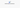# JavaScript merge array of objects by key (es6)In this tutorial, we are going to learn about how to merge an array of objects by using key in JavaScript.

Suppose we have two array of objects with the same key.

Example:

``````const arr1 =[
{id:1,name:"sai"},
{id:2,name: "King"}
];
const arr2 = [
{id:1,age:23},
{id:2,age:24}
];``````

Now we need to merge the two array of objects into a single array by using `id` property because `id` is the same in both array objects.

Note: Both arrays should be the same length to get a correct answer.

## First way

Here we are the using map method and Object.assign method to merge the array of objects by using `id`.

``````function mergeArrayObjects(arr1,arr2){
return arr1.map((item,i)=>{
if(item.id === arr2[i].id){
//merging two objects
return Object.assign({},item,arr2[i])
}
})
}

console.log(mergeArrayObjects(arr1,arr2));

/* output
[
{id: 1, name: "sai", age: 23},
{id: 2, name: "King", age: 24}
]
*/``````## Second way

In this example, we are using `while` loop to loop over through the array of objects and merging the objects using `spread` operator.

``````function mergeArrayObjects(arr1,arr2){
let start = 0;
let merge = [];

while(start < arr1.length){
if(arr1[start].id === arr2[start].id){
//pushing the merged objects into array
merge.push({...arr1[start],...arr2[start]})    }
//incrementing start value
start = start+1
}
return merge;
}``````